CAT  >  Examples : Slope of a Line & Straight Line

# Examples : Slope of a Line & Straight Line Notes | Study Quantitative Aptitude (Quant) - CAT

## Document Description: Examples : Slope of a Line & Straight Line for CAT 2022 is part of Quantitative Aptitude (Quant) preparation. The notes and questions for Examples : Slope of a Line & Straight Line have been prepared according to the CAT exam syllabus. Information about Examples : Slope of a Line & Straight Line covers topics like and Examples : Slope of a Line & Straight Line Example, for CAT 2022 Exam. Find important definitions, questions, notes, meanings, examples, exercises and tests below for Examples : Slope of a Line & Straight Line.

Introduction of Examples : Slope of a Line & Straight Line in English is available as part of our Quantitative Aptitude (Quant) for CAT & Examples : Slope of a Line & Straight Line in Hindi for Quantitative Aptitude (Quant) course. Download more important topics related with notes, lectures and mock test series for CAT Exam by signing up for free. CAT: Examples : Slope of a Line & Straight Line Notes | Study Quantitative Aptitude (Quant) - CAT
 1 Crore+ students have signed up on EduRev. Have you?

Slope of a line

First, talk in intuitive terms about what is meant by slope. Give real-life examples of slope such as the slope of
the roof of a house, a road going up a hill, or a ladder leaning against a building. Explain that we can assign a
number that allows us to measure the steepness of a straight line. Also, say that the greater the absolute
value of this number, the steeper the line will be.

Slope of a non-vertical line L is the tangent of the angle θ, which the line L makes with the positive direction of
x-axis. In particular,

(a) Slope of a line parallel of x-axis is zero.

(b) Slope of a line parallel to y-axis is not defined.

(c) Slope of a line equally inclined to the axis is −1 or 1.

(d) Slope of a line making equal intercepts on the axis is −1.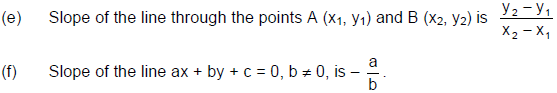(g) Slopes of two parallel (non-vertical) lines are equal. If m1, m2 are the slopes, then m1 = m2.

(h) If m1 and m2 be the slopes of two perpendicular lines (which are oblique), then m1m2 = - 1.

Straight line

Straight-line equations, or "linear" equations, graph as straight lines, and have simple variables with no
exponents on them. If you see an equation with x and y, then you're dealing with a straight-line equation.

An equation of the form ax + by + c = 0 is called the general equation of a straight line, where x and y are
variable and a, b, c are constants.

Equation of a line parallel to X axis or Y - axis

(i) Equation of any line parallel to x-axis is y = b, b being the directed distance
of the line from the x-axis. In particular equation of x-axis is y = 0

(ii) Equation of any line parallel to y-axis is x = a, a being the directed distance
of the line from the y-axis. In particular equation of y-axis is x = 0.

(a) One point form

Equation of a line (non-vertical) through the point (x1, y1) and having
slope m is
y - y1 = m (x - x1).

(b) Two-point form

Equation of a line (non-vertical) through the points (x1, y1) and (x2, y2) is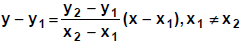(c) Slope-intercept form

Equation of a line (non-vertical) with slope m and cutting off an intercept c from the y-axis is
y = m x + c.

(d) Intercept form

Equation of a line (non-vertical) with slope m and cutting off intercepts a and b from the x-axis
and y-axis respectively is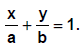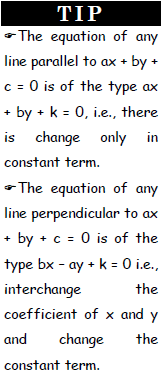Ex.1 Line intersects x axis at A (10, 0) and y-axis at B (0, 10). Find the equation of the line.
(1) x + y = 10

(2) x + y = 20

(3) x = - y

(4) None of these

Sol. As line intersects x-axis at A (10, 0)
⇒ length of intercept on x-axis, a = 10
Similarly length of intercept on y-axis, b = 10
∴ Using intercept form, equation of line is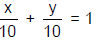or x + y = 10. Answer: (1)

Ex.2 Find the equation of the straight line passing through the point (- 2, - 3) and perpendicular to
the line through (- 2, 3) and (- 5, - 6).
(1) X + 2 Y + 8 = 0

(2) X + 3Y + 11 = 0

(3) X - 3Y = 7

(4) X + 3Y = 11

Sol. The slope of the line through (- 2, 3) and (- 5, - 6) is m =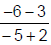= 3

⇒ The slope m1 of the required line =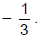By point - slope form, Y + 3 =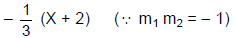⇒ X + 3Y + 11 = 0. Answer: (2)

Ex.3 Find the slope of the line passing through (- 3, 7) having Y-intercept - 2.
(1) - 5

(2) 2

(3) - 3

(4)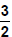Sol. The line passes through the points (- 3, 7) and (0, - 2).
∴ Slope of the line =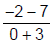= - 3. Answer: (3)

Some Important Results
• Length of perpendicular from the point (x1, y1) to the line ax + by + c = 0 is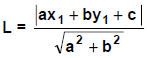• Distance between parallel lines ax + by + c = 0 and ax + by + d = 0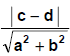• The angle between two lines y = m1x + b1 and y = m2x + b2 is given by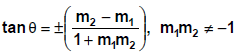• The equation a1x + b1y + c1 = 0 and a2x + b2y + c2 = 0 represent the same line if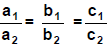Concurrent Lines:

Three or more lines are said to be concurrent lines when all of them pass through a common point.

The document Examples : Slope of a Line & Straight Line Notes | Study Quantitative Aptitude (Quant) - CAT is a part of the CAT Course Quantitative Aptitude (Quant).
All you need of CAT at this link: CAT

## Quantitative Aptitude (Quant)

163 videos|164 docs|131 tests
 Use Code STAYHOME200 and get INR 200 additional OFF

## Quantitative Aptitude (Quant)

163 videos|164 docs|131 tests

### How to Prepare for CAT

Read our guide to prepare for CAT which is created by Toppers & the best Teachers

Track your progress, build streaks, highlight & save important lessons and more!

,

,

,

,

,

,

,

,

,

,

,

,

,

,

,

,

,

,

,

,

,

;